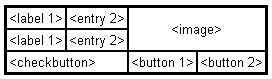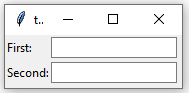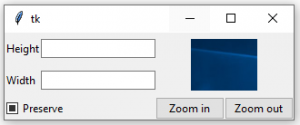# Python – grid() method in Tkinter

## Python | grid() method in Tkinter

The Grid geometry manager puts the widgets in a 2-dimensional table. The master widget is split into a number of rows and columns, and each “cell” in the resulting table can hold a widget.
The grid manager is the most flexible of the geometry managers in Tkinter. If you don’t want to learn how and when to use all three managers, you should at least make sure to learn this one.

Consider the following example –Creating this layout using the pack manager is possible, but it takes a number of extra frame widgets, and a lot of work to make things look good. If you use the grid manager instead, you only need one call per widget to get everything laid out properly.

Using the grid manager is easy. Just create the widgets, and use the grid method to tell the manager in which row and column to place them. You don’t have to specify the size of the grid beforehand; the manager automatically determines that from the widgets in it.

Code #1:

 `# import tkinter module` `from` `tkinter ``import` `*` `from` `tkinter.ttk ``import` `*` ` ` `# creating main tkinter window/toplevel` `master ``=` `Tk()` ` ` `# this wil create a label widget` `l1 ``=` `Label(master, text ``=` `"First:"``)` `l2 ``=` `Label(master, text ``=` `"Second:"``)` ` ` `# grid method to arrange labels in respective` `# rows and columns as specified` `l1.grid(row ``=` `0``, column ``=` `0``, sticky ``=` `W, pady ``=` `2``)` `l2.grid(row ``=` `1``, column ``=` `0``, sticky ``=` `W, pady ``=` `2``)` ` ` `# entry widgets, used to take entry from user` `e1 ``=` `Entry(master)` `e2 ``=` `Entry(master)` ` ` `# this will arrange entry widgets` `e1.grid(row ``=` `0``, column ``=` `1``, pady ``=` `2``)` `e2.grid(row ``=` `1``, column ``=` `1``, pady ``=` `2``)` ` ` `# infinite loop which can be terminated by keyboard` `# or mouse interrupt` `mainloop()`

Output:Code #2: Creating the layout which is shown above.

 `# import tkinter module` `from` `tkinter ``import` `*` `from` `tkinter.ttk ``import` `*` ` ` `# creating main tkinter window/toplevel` `master ``=` `Tk()` ` ` `# this will create a label widget` `l1 ``=` `Label(master, text ``=` `"Height"``)` `l2 ``=` `Label(master, text ``=` `"Width"``)` ` ` `# grid method to arrange labels in respective` `# rows and columns as specified` `l1.grid(row ``=` `0``, column ``=` `0``, sticky ``=` `W, pady ``=` `2``)` `l2.grid(row ``=` `1``, column ``=` `0``, sticky ``=` `W, pady ``=` `2``)` ` ` `# entry widgets, used to take entry from user` `e1 ``=` `Entry(master)` `e2 ``=` `Entry(master)` ` ` `# this will arrange entry widgets` `e1.grid(row ``=` `0``, column ``=` `1``, pady ``=` `2``)` `e2.grid(row ``=` `1``, column ``=` `1``, pady ``=` `2``)` ` ` `# checkbutton widget` `c1 ``=` `Checkbutton(master, text ``=` `"Preserve"``)` `c1.grid(row ``=` `2``, column ``=` `0``, sticky ``=` `W, columnspan ``=` `2``)` ` ` `# adding image (remember image should be PNG and not JPG)` `img ``=` `PhotoImage(``file` `=` `r``"C:\Users\Admin\Pictures\capture1.png"``)` `img1 ``=` `img.subsample(``2``, ``2``)` ` ` `# setting image with the help of label` `Label(master, image ``=` `img1).grid(row ``=` `0``, column ``=` `2``,` `       ``columnspan ``=` `2``, rowspan ``=` `2``, padx ``=` `5``, pady ``=` `5``)` ` ` `# button widget` `b1 ``=` `Button(master, text ``=` `"Zoom in"``)` `b2 ``=` `Button(master, text ``=` `"Zoom out"``)` ` ` `# arranging button widgets` `b1.grid(row ``=` `2``, column ``=` `2``, sticky ``=` `E)` `b2.grid(row ``=` `2``, column ``=` `3``, sticky ``=` `E)` ` ` `# infinite loop which can be terminated ` `# by keyboard or mouse interrupt` `mainloop()`

Output:Warning: Never mix grid() and pack() in the same master window.

Last Updated on October 24, 2021 by admin

## Python DestructorsPython Destructors

Destructors in Python Destructors are called when an object gets destroyed. In Python, destructors are

## Python – Key index in DictionaryPython – Key index in Dictionary

Python | Key index in Dictionary The concept of dictionary is similar to that of

Python | Read csv using pandas.read_csv() Python is a great language for doing data analysis,

## Python Nested DictionaryPython Nested Dictionary

Python Nested Dictionary A Dictionary in Python works similar to the Dictionary in the real

## SSL Certificate Verification – Python requestsSSL Certificate Verification – Python requests

SSL Certificate Verification – Python requests Requests verifies SSL certificates for HTTPS requests, just like

## Pandas dataframe.set_value()Pandas dataframe.set_value()

Pandas dataframe.set_value()   Pandas dataframe.set_value() function put a single value at passed column and index.

## Python | Maximum and minimum element’s position in a listPython | Maximum and minimum element’s position in a list

Python – Maximum and minimum element’s position in a list   Given a list of

## Python – Check if dictionary is emptyPython – Check if dictionary is empty

Python | Check if dictionary is empty Sometimes, we need to check if a particular